Courses

# Test: Index Numbers- 3

## 40 Questions MCQ Test Quantitative Aptitude for CA CPT | Test: Index Numbers- 3

Description
This mock test of Test: Index Numbers- 3 for CA Foundation helps you for every CA Foundation entrance exam. This contains 40 Multiple Choice Questions for CA Foundation Test: Index Numbers- 3 (mcq) to study with solutions a complete question bank. The solved questions answers in this Test: Index Numbers- 3 quiz give you a good mix of easy questions and tough questions. CA Foundation students definitely take this Test: Index Numbers- 3 exercise for a better result in the exam. You can find other Test: Index Numbers- 3 extra questions, long questions & short questions for CA Foundation on EduRev as well by searching above.
QUESTION: 1

Solution:

QUESTION: 2

Solution:
QUESTION: 3

### Circular Test satisfy is

Solution:
QUESTION: 4

From the following data for the 5 groups combined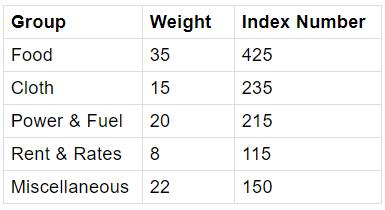The general Index number is

Solution:

Explanation :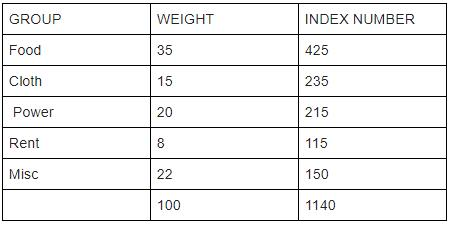Group weight index number  =  Weighted Index/ Total Weight

=  26920/100

= 269.2

Group weight index number = 269.2

QUESTION: 5

From the following data with 1966 as base year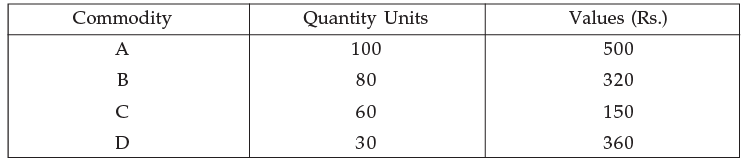Q. The price per unit of commodity A in 1966 is

Solution:
QUESTION: 6

The index number in whole sale prices is 152 for August 1999 compared to August 1998.During the year there is net increase in prices of whole sale commodities to the extent of

Solution:
QUESTION: 7

The value Index is expressed in terms of

Solution:
QUESTION: 8

Solution:
QUESTION: 9

The price level of a country in a certain year has increased 25% over the base period.The index number is

Solution:
QUESTION: 10

The index number of prices at a place in 1998 is 355 with 1991 as base. This means

Solution:
QUESTION: 11

If the price of all commodities in a place have increased 125 times in comparison to the base period prices, then the index number of prices for the place is now

Solution:
QUESTION: 12

The whole sale price index number or agricultural commodities in a given region at a given date is 280. The percentage use in prices of agricultural commodities over the base year is :

Solution:
QUESTION: 13

If now the prices of all the commodities in a place have been decreased by 85% over the base period prices, then the index number of prices for the place is now (index number of prices of base period = 100)

Solution:
QUESTION: 14

From the data given below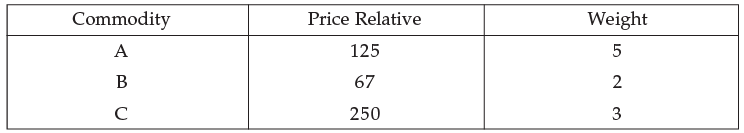Q. Then the suitable index number is

Solution:
QUESTION: 15

Bowley’s Index number is expressed in terms of :

Solution:
QUESTION: 16

From the following data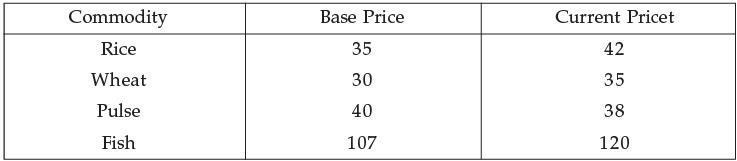Q. The simple Aggregative Index is

Solution:
QUESTION: 17

With regard to Laspeyre’s and Paasche’s price index number, it is maintained that “If the prices of all the goods change in the same ratio, the two indices will be equal for them the weighting system is irrelevant; or if the quantities of all the goods change in the same ratio, they will be equal, for them the two weighting systems are the same relatively”.Then the above statements satisfy.

Solution:
QUESTION: 18

The quantity Index number using Fisher’s formula satisfies :

Solution:
QUESTION: 19

For constructing consumer price Index is used :

Solution:
QUESTION: 20

The cost of living Index (C.L.I.) is always :

Solution:
QUESTION: 21

The Time Reversal Test is not satisfied to :

Solution:
QUESTION: 22

Given below are the date on prices of some consumer goods and the weights attached to the various items Compute price index number for the year 1985 (Base 1984 = 100)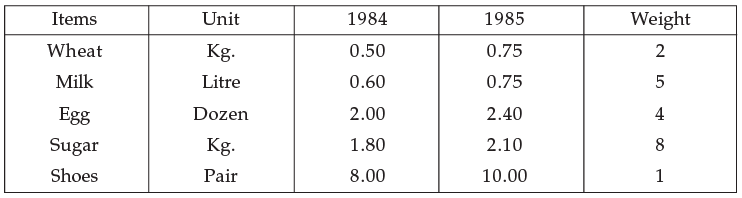Q. Then weighted average of price Relative Index is :

Solution:
QUESTION: 23

The Factor Reversal Test is as represented symbolically is :

Solution:
QUESTION: 24

If the 1970 index with base 1965 is 200 and 1965 index with base 1960 is 150, the index 1970 on base 1960 will be :

Solution:

Then  1965 index with base 1960 is  150

=>  1960 index  = 100

the 1970 index with base 1965 is 200

=> 1965 index  = 100

When 1965 index = 100 then  1970 index = 200

When 1965 index = 1 then  1970 index = 200/100

When 1965 index = 150 then  1970 index = (200/100) * 150 = 300

so 1970 index = 300   When 1960 index = 100

Hence the index 1970 on the base 1960 will be​ 300

QUESTION: 25

Circular Test is not met by :

Solution:
QUESTION: 26

From the following data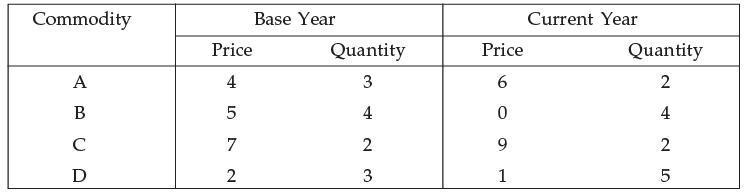Q. Then the Factor Reversal Test is :

Solution:
QUESTION: 27

The value index is equal to :The value index is equal to :

Solution:
QUESTION: 28

Time Reversal Test is represented symbolically by :

Solution:
QUESTION: 29

In 1996 the average price of a commodity was 20% more than in 1995 but 20% less than in 1994; and more over it was 50% more than in 1997 to price relatives using 1995 as base (1995 price relative 100) Reduce the data is :

Solution:
QUESTION: 30

From the following data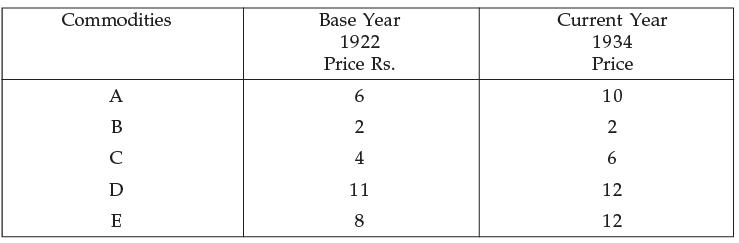Q. The price index number for the year 1934 is :

Solution:

Solution :- Price Index = ΣP(Current area)/ΣP(Base Area) * 100

= (42/31)*100

= 135.48

QUESTION: 31

From the following data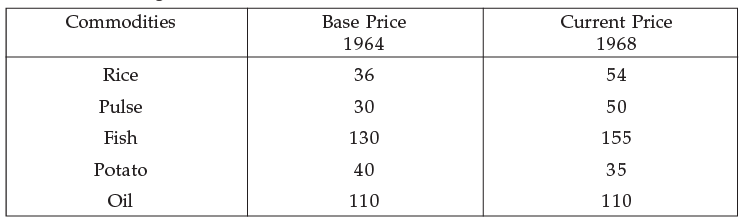Q. The index number by unweighted methods :

Solution:
QUESTION: 32

The Bowley’s Price index number is represented in terms of :

Solution:
QUESTION: 33

Fisher’s price index number equal is :

Solution:
QUESTION: 34

The price index number using simple G.M. of the relatives is given by :

Solution:
QUESTION: 35

The price of a number of commodities are given below in the current year 1975 and base year 1970.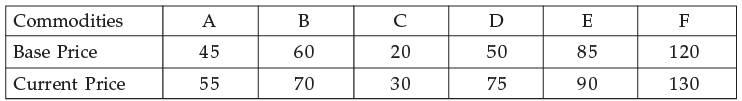Q. For 1975 with base 1970 by the Method of price relatives using Geometrical mean. The price index is :

Solution:
QUESTION: 36

From the following data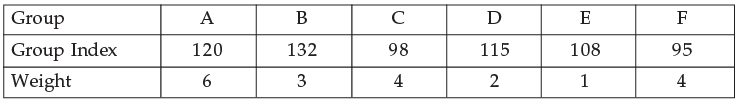Q. The general Index I is given by :

Solution:
QUESTION: 37

The price of a commodity increases from Rs. 5 per unit in 1990 to Rs. 7.50 per unit in 1995 and the quantity consumed decreases from 120 units in 1990 to 90 units in 1995. The price and quantity in 1995 are 150% and 75% respectively of the corresponding price and quantity in 1990. Therefore, the product of the price ratio and quantity ratio is :

Solution:
QUESTION: 38

Test whether the index number due to Walsh give by :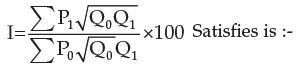Solution:
QUESTION: 39

From the following data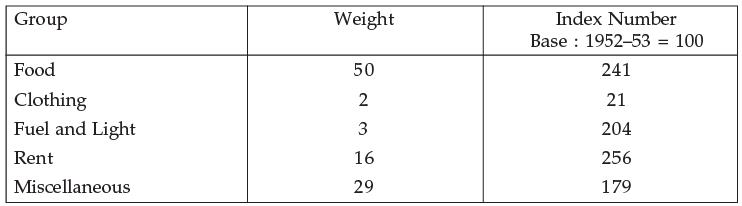Q. The Cost of living index numbers is :

Solution:

QUESTION: 40

Consumer price index number goes up from 110 to 200 and the Salary of a worker is also raised from Rs. 325 to Rs. 500. Therefore, in real terms he has not gain, to maintain his previous standard of living he should get an additional amount is :

Solution: